Courses

# Feedback Amplifiers

## 10 Questions MCQ Test Topicwise Question Bank for Electronics Engineering | Feedback Amplifiers

Description
This mock test of Feedback Amplifiers for Electronics and Communication Engineering (ECE) helps you for every Electronics and Communication Engineering (ECE) entrance exam. This contains 10 Multiple Choice Questions for Electronics and Communication Engineering (ECE) Feedback Amplifiers (mcq) to study with solutions a complete question bank. The solved questions answers in this Feedback Amplifiers quiz give you a good mix of easy questions and tough questions. Electronics and Communication Engineering (ECE) students definitely take this Feedback Amplifiers exercise for a better result in the exam. You can find other Feedback Amplifiers extra questions, long questions & short questions for Electronics and Communication Engineering (ECE) on EduRev as well by searching above.
QUESTION: 1

### When an amplifier is provided with current-shunt feedback, its

Solution: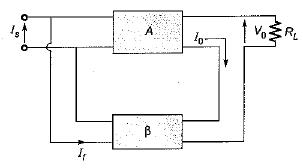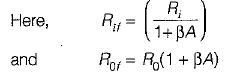QUESTION: 2

### An amplifier has an open-loop gain of 1000 and a feedback ratio of 0.04. If the open-loop gain changes by 10% due to temperature, the percentage change in gain of the amplifier with feedback will be

Solution:

Given,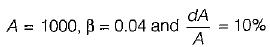The percentage changeing a in of the amplifier with feed back is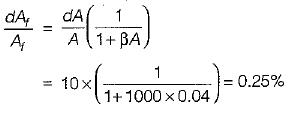QUESTION: 3

### A voltage-series negative feedback amplifier has a voltage gain without feedback of A = 500, input resistance R( = 3 kΩ and feedback ratio p = 0.01. The voltage gain Af and input resistance Rtf of the amplifier with feedback are respectively

Solution: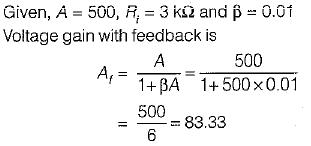For a voltage-series negative feedback amplifier, input resistance with negative feedback is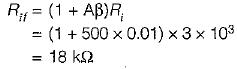QUESTION: 4

Assertion (A): The emitter follower inherently exhibits 100% negative feedback.
Reason (R): The emitter follower is an example of voltage-series feedback.

Solution:

The emitter follower inherently exhibits 100% negative feedback because the voltage at the emitter follows the input voltage. Reason is also a correct statement since the emitter follower simultaneously increases input resistance and decreases output resistance. Hence, both assertion and reason are correct but reason is not the correct explanation of assertion.

QUESTION: 5

For the emitter follower circuit shown below, match List-l with List-ll and select the correct answer using the codes given below the lists: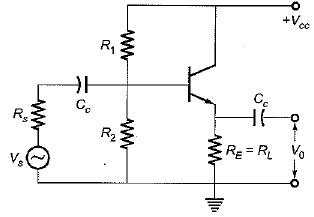List-I
A.    Voltage gain (AV)
B.    input resistance (Ri)
C.    Current gain (Ai)
D.    Output resistance (without feedback)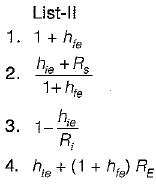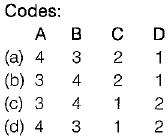Solution:
QUESTION: 6

An amplifier has a mid-band gain of 125 and a bandwidth of 250 kHz. A 4% negative feedback is introduced to obtain a new bandwidth and gain. If the bandwidth is to be restricted to 1 MHz, the feedback ratio will be

Solution:

Due to negative feedback, bandwidth becomes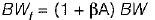Given, BW = 250 kHz
A = 125, BWf = 1 MHz
Let β’ be the feedback ratio required to restrict the bandwidth to 1 MHz.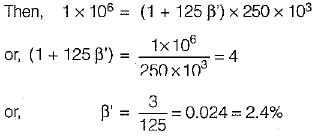QUESTION: 7

An amplifier has voltage gain with feedback of 100. If the gain without feedback changes by 20% and the gain with feedback should not vary more than 2%, the values of open loop gain and feedback ratio will be respectively given by

Solution:

We know that,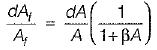or,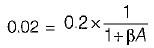or,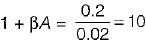Also, gain with feedback is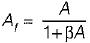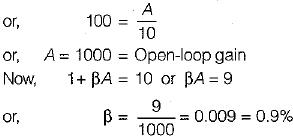QUESTION: 8

Match List-l (Type of negative feedback) with List-II (Common names) and select the correct answer using the codes given below the lists:
List-l
A. Voltage-shunt feedback
B. Current-series feedback
C. Voltage-series feedback
D. Current-shunt feedback
List-ll
1. Trans-conductance amplifier
2. Current amplifier
3. Trans-resistance amplifier
4. Voltage amplifier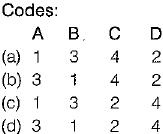Solution:
QUESTION: 9

Negative feedback enhances all performance parameters of an amplifier except its

Solution:

Due to negative feedback, gain of the amplifier is reduced
i.e.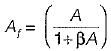QUESTION: 10

Shunt mixing is generally easy to identify because it

Solution: